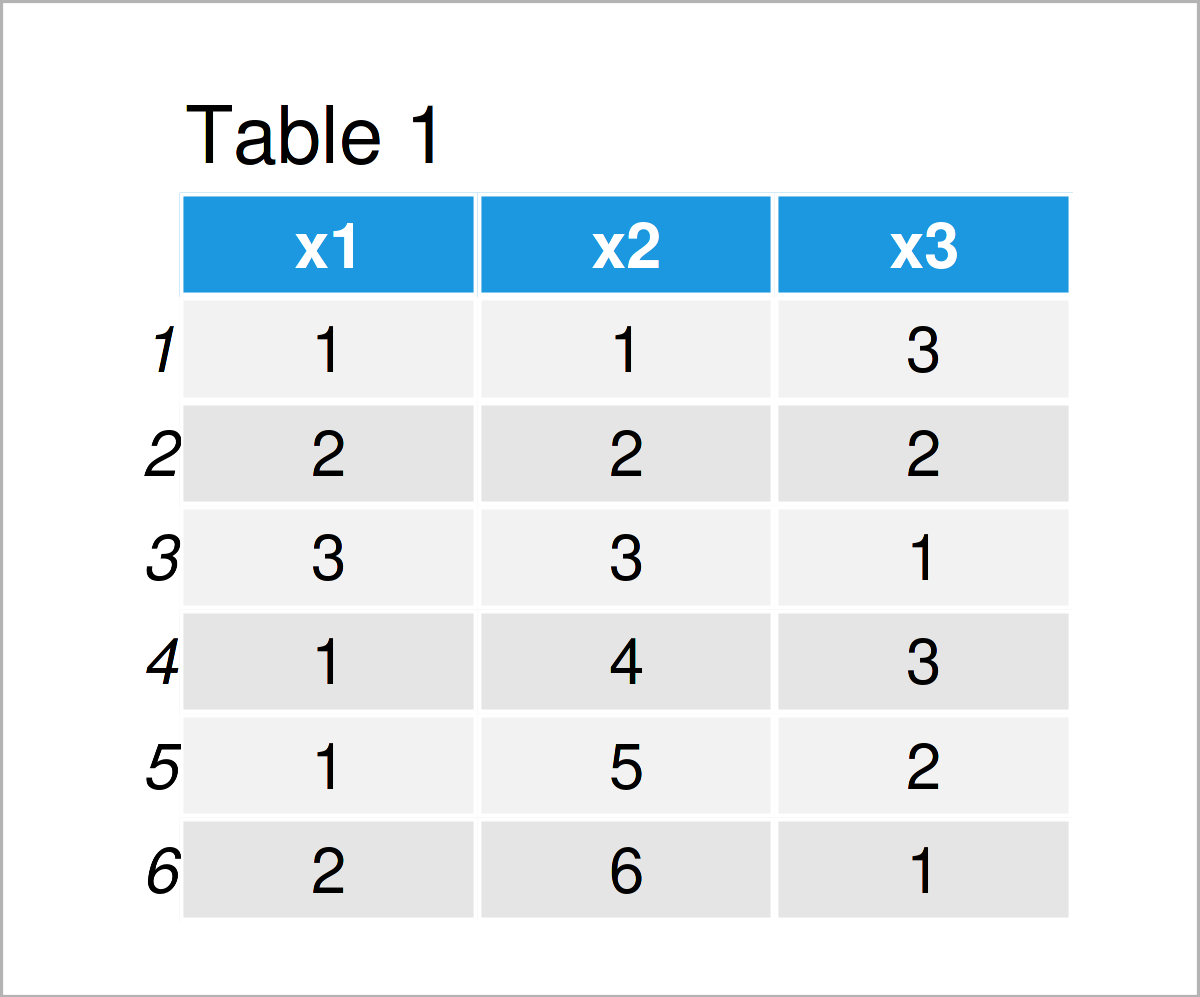# Replace Multiple Values in Several Columns of Data Frame in R (2 Examples)

In this post, I’ll show how to exchange multiple values in certain data frame columns in R.

Let’s get started.

## Example Data

Let’s first define some example data:

```data <- data.frame(x1 = c(1, 2, 3, 1, 1, 2),        # Create example data
x2 = 1:6,
x3 = 3:1)
data                                                # Print example data```Have a look at the table that has been returned after executing the previous R code. It shows that our example data is composed of six data points and three columns. The variable x1 is numerical and the variables x2 and x3 have the integer class.

Next, we have to specify a vector of values that we want to replace in our data frame:

```val_repl <- c(1, 3)                                 # Specify values to be replaced
val_repl                                            # Print vector of values
#  1 3```

As you can see, we have specified that we want to replace the numbers 1 and 3.

Let’s do this!

## Example 1: Replace Multiple Values in All Columns of Data Frame

In Example 1, I’ll demonstrate how to conditionally replace certain values in all variables of a data frame.

For this task, we can use the sapply and replace functions as shown below:

```data_new1 <- sapply(data,                           # Replace values in all columns
function(x) replace(x, x %in% val_repl, 99))
data_new1                                           # Print updated data frame```As shown in Table 2, we have created a new data frame where all values equal to 1 or 3 have been replaced by the new value 99.

## Example 2: Replace Multiple Values in Particular Columns of Data Frame

Example 2 explains how to replace values only in specific columns of a data frame.

For this, we first have to specify the columns we want to change:

```col_repl <- c("x2", "x3")                           # Specify columns
col_repl                                            # Print vector of columns
#  "x2" "x3"```

Next, we can use the R syntax below to modify the selected columns, i.e. x2 and x3:

```data_new2 <- data                                   # Duplicate data frame
data_new2[col_repl] <- sapply(data_new2[col_repl],  # Replace values in certain columns
function(x) replace(x, x %in% val_repl, 99))
data_new2                                           # Print updated data frame```By running the previous R syntax, we have created Table 3, i.e. another updated version of our input data frame where only the variables x2 and x3 have been substituted.

## Video & Further Resources

In case you need further explanations on the R programming code of this article, you may have a look at the following video on my YouTube channel. I’m explaining the content of this post in the video.

Furthermore, you may want to have a look at the related articles on this website.

In this article you have learned how to exchange multiple values in certain data frame variables in R. Please let me know in the comments below, if you have additional questions.

Subscribe to the Statistics Globe Newsletter